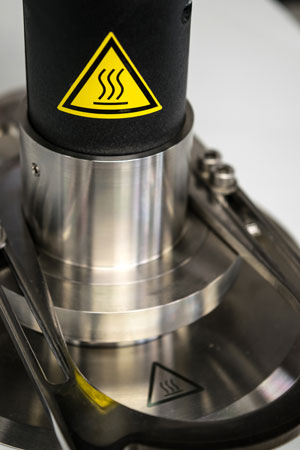The thermo-gravimetric analysis (TGA) is a method of thermal analysis in which changes in physical and chemical properties of materials are measured as a function of increasing temperature.

TGA is commonly used to determine selected characteristics of materials that exhibit either mass loss or gain due to decomposition, oxidation, or loss of volatiles (such as moisture). Common applications of TGA are: materials characterization through analysis of characteristic decomposition patterns, studies of degradation mechanisms and reaction kinetics, determination of organic content in a sample, and determination of inorganic (e.g. ash) content in a sample, which may be useful for corroborating predicted material structures or simply used as a chemical analysis.

Differential scanning calorimetry (DSC) is also a thermoanalytical technique in which the difference in the amount of heat required to increase the temperature of a sample and reference is measured as a function of temperature. Both the sample and reference are maintained at nearly the same temperature throughout the experiment. The DSC allows precise measurements of heat capacity and can be also used for calculation of enthalpies of chemical reactions.